# Lateral Buckling of Narrow Rectangular BeamThis test lets you check analysis results for rectangular beams, in the context of a buckling case.
You will use 1D meshes.Reference:

• W. FLIGE, MC GRAW-HILL, Handbook of Engineering Mechanics, New York, 1962

• J.H. ARGYRIS, Finite Element Method - The Natural Approach, 1979

##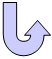Specifications

### Geometry Specifications

 Length: L1 = 240 mm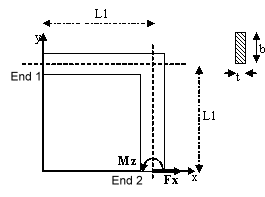Height: b = 30 mm Thickness: t = 0.6 mm

### Analysis Specifications

 Young Modulus (material): E = 71240 GPa Poisson's Ratio (material): ν = 0.31 Inertia: Ix = 2.16 mm4 (torsionnal) Iy = 0.54 mm4 (minor) Iz = 1350 mm4 (major) Mesh Specifications: 21 nodes, 20 beam elements. Restraints (User-defined): Test 1: At End 1, all degrees of freedom for nodes are fixed  Test 2: At End 1, Tx = Ty = Tz = Rx = Ry = 0 At End 2, Ty = Tz = Rx = Ry = 0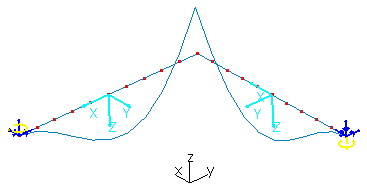Loads: Test 1: At End 2, distributed force Fx = 1 N Test 2: At End 1, moment Mz = -1 N x mm At End 2, moment Mz = 1 N x mm

##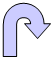Results

The following table presents the results for buckling eigen values.

 Argyris theory CATIA beam Test 1 1.088 1.088 Test 2 624.77 624.77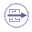To Perform the Test:

The Lateral_buckling_rectangular_beam.CATAnalysis document presents the complete lateral buckling case, and includes the tests 1 and 2.

To compute the case, proceed as follow:

1. Open the CATAnalysis document.

2. Compute the analysis and generate an image called Deformed Mesh.